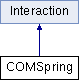ParM  parm A molecular dynamics library
COMSpring Class Reference

#include <interaction.hpp>

Inheritance diagram for COMSpring:## Public Member Functions

COMSpring (AtomGroup *g1, AtomGroup *g2, flt k, flt x0=0)

flt energy (Box &box)
Potential energy due to this Interaction. More...

void set_forces (Box &box)

flt pressure (Box &box)
Partial pressure due to this Interaction. More...Public Member Functions inherited from Interaction
virtual flt set_forces_get_pressure (Box &box)
Set forces (Atom.f) and return $$P = \sum_{\left<i,j \right>} \vec r_{ij} \cdot \vec F_{ij}$$ at the same time (see pressure()). More...

virtual Matrix stress (Box &box)
The force-moment tensor for the current simulation: More...

virtual ~Interaction ()

AtomGroupg1

AtomGroupg2

flt k

flt x0

flt m1

flt m2

## Constructor & Destructor Documentation

 COMSpring::COMSpring ( AtomGroup * g1, AtomGroup * g2, flt k, flt x0 = 0 )
inline

## Member Function Documentation

 flt COMSpring::energy ( Box & box )
inlinevirtual

Potential energy due to this Interaction.

Implements Interaction.

 flt COMSpring::pressure ( Box & box )
inlinevirtual

Partial pressure due to this Interaction.

$$P = \sum_{\left<i,j \right>} \vec r_{ij} \cdot \vec F_{ij}$$, or equivalently $$P = \sum_i \vec r_i \cdot \vec F_i$$

Note that the full pressure involves all interactions and temperature, and needs to be normalized by $$\frac{1}{dV}$$ where $$d$$ is the number of dimensions and $$V$$ is the volume.

Implements Interaction.

 void COMSpring::set_forces ( Box & box )
inlinevirtual

Implements Interaction.

## Member Data Documentation

 AtomGroup* COMSpring::g1
protected
 AtomGroup* COMSpring::g2
protected
 flt COMSpring::k
protected
 flt COMSpring::m1
protected
 flt COMSpring::m2
protected
 flt COMSpring::x0
protected

The documentation for this class was generated from the following file: